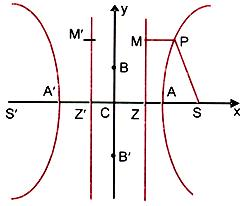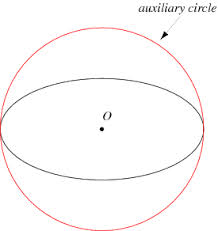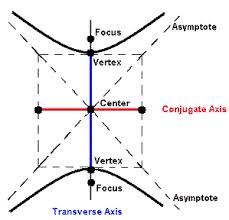×#### Thank you for registering.

One of our academic counsellors will contact you within 1 working day.

Click to Chat

1800-1023-196

+91-120-4616500

CART 0

• 0

MY CART (5)

Use Coupon: CART20 and get 20% off on all online Study Material

ITEM
DETAILS
MRP
DISCOUNT
FINAL PRICE
Total Price: Rs.

There are no items in this cart.
Continue Shopping```Basic Concepts of Hyperbola

Table of Content

Standard Equation

Terms Related to Hyperbola

Important facts

Auxiliary Circle

Conjugate Hyperbola

Related ResourcesA hyperbola is the locus of a point which moves in a plane in such a way that the ratio of its distance from a fixed point called as focus and a fixed line called the directrix is a constant (called ‘e’ the eccentricity) which is greater than 1. Thus, for hyperbola, the eccentricity e > 1.

A hyperbola can be obtained by slicing a right circular cone at both the nappes by a plane. Thus, a hyperbola can be assumed to be a single curve having two branches, one on each nappe.

Standard Equation

Let S be the focus and ZM the directrix of a hyperbola.Since e > 1, we can divide SZ internally and externally in the ratio e : 1; let the points of division be A and A’ as in the figure. Let AA’ = 2a and be bisected at C. Then, SA = e.AZ, SA’ = e.ZA’

⇒ SA + SA’ = e(AZ + ZA’) = 2ae

i.e., 2SC = 2ae or SC = ae.

Similarly by subtraction, SA’ – SA

= e(ZA’ – ZA) = 2e.ZC ⇒ 2a = 2eSC ⇒ SC = a/e.

Now, take C as the origin, CS as the x-axis, and the perpendicular line CY as the y-axis. Then, S is the point (ae, 0) and ZM the line x = a/e. Let P(x, y) be any point on the hyperbola. Then the condition PS2 = e2.(distance of P from ZM)2 gives (x – ae)2 + y2 = e2 (x – a/e)2 or x2(1 – e)2+ y2 = a2(1 – e2)

i.e. x2/a2 – y2/a2(e2–1) = 1.                                      …… (i)

Since e > 1, e2 – 1 is positive. Let a2 (e2 – 1) = b2. Then the equation (i) becomes x2/a2 – y2/a2 = 1.

The eccentricity e of the hyperbola x2/a2 – y2/a2 = 1 is given by the relation e2 = (1 + b2/a2).

Since the curve is symmetrical about the y-axis, it is clear that there exists another focus S’ at (–ae, 0) and a corresponding directrix Z’M’ with the equation x = –a/e, such that the same hyperbola is described if a point moves so that its distance from S’ is e times its distance from Z’M’.

Terms Related to Hyperbola

Symmetry:

Since only even powers of x and y occur in the above equation, hence the curve is symmetrical about both the axis.

Foci:

S and S’ are the two foci of the hyperbola and their coordinates are (ae, 0) and (-ae, 0) respectively. the distance between the foci is given by SS’ = 2ae.

Directrices:

ZM and Z’M’ are the two directirces of the hyperbola and their equations are x = a/e and x = -a/e respectively. The distance between the directrices is given by ZZ’ = 2a/e.

Axes:

The lines AA’ and BB’ are called the transverse axis and conjugate axis respectively of teh hyperbola. the length of the transverse axis is AA’ = 2a. Similarly, teh length of conjugate axis is BB’ = 2b.

Centre:

The point of intersection of the axes of the hyperbola is called as the centre of the hyperbola.

Vertices:

The point A(a, 0) and A’(-a, 0) where the curve meets the line joining the foci S and S’ are called the vertices of the hyperbola.

Focal Chord:

A chord of the hyperbola passing through its focus is called the focal chord.

Focal Distance:

The focal distance of any point (x, y) on teh hyperbola x2/a2 – y2/b2 = 1 are given by (ex-a) and (ex+a).

Latus Rectum:

If LL’ and NN’ are the latus rectum of the hyperbola tehn these lines are perpendicular to the transverse axis AA’ passing through the foci S and S’ respectively.

Then L = (ae, b2/a) , L’ = (ae, -b2/a) , N = (-ae, b2/a) , N’ = (-ae, -b2/a).

Hence, the length of latus rectum = LL’ = 2b2/a = NN’.

A latus rectum is thus the chord through a focus at right angle to the transverse axis.Important facts

All chords passing through the centre C are bisected at C.

Proof: It can be proved by taking P(x1, y1) as any point on the hyperbola. If (x1, y1) lies on the hyperbola then so does P(–x1, –y1) because the hyperbola is symmetrical about the x and the y axes. Therefore PP’ is a chord whose middle point (0, 0), i.e. the origin C. On account of this property the middle point of the straight line joining the vertices of the hyperbola is called the centre of the hyperbola.

The length of the semi-latus rectum can be obtained by putting x = ae in the equation of the hyperbola. Thus y = b √a2e2/a2–1 = b√e2–1 = b.b/a = b2/a.

The most general equation of hyperbola whose focus is the point (h, k), directrix is lx + my + n = 0 and the eccentricity ‘e’ is given by (x-h)2 + (y-k)2 = e2
(lx + my + n)2/(l2 + m2)

Since the coordinate x = a sec θ and y = b tan θ satisfy the standard equation x2/a2 – y2/b2 = 1 for all real values of θ, therefore x = a sec θ and y = b tan θ are the parametric equations of the hyperbola x2/a2 – y2/b2 = 1, where the parameter θ lies between 0 ≤ θ ≤ 2π.

Auxiliary Circle

A cricle drawn with centre C and transverse axis as a diameter is called the auxiliary circle of the hyperbola. The equation of the auxiliary circle is x2 + y2 = a2.Conjugate Hyperbola

The hyperbola whose transverse and conjugate axes are respectively the conjugate and transverse axes of a given hyperbola is called the conjugate hyperbola of the given hyperbola. The conjugate hyperbola is therefore of the formThe standard equation of the conjugate hyperbola of the hyperbola  x2/a2 – y2/b2 = 1 is  x2/a2 – y2/b2 = -1.

Students are advised to refer the coming sections to study these topics in detail.

Illustration:

The line 2x + y = 1 is tangent to the hyperbola x2/a2 – y2/b2 = 1. If this line passes through the point of intersection of teh nearest directrix and the x-axis then the eccentricity of the hyperbola is ? (IIT JEE 2010)

Solution:

We substitute (a/e, 0) in the equation y = -2x + 1, we get

0 = -2a/e + 1

This gives a/e = 1/2

Also, y = -2x + 1 is tangent to hyperbola

Hence, 1 = 4a2 – b2

Hence, 1/a2 = 4 – (e2 – 1)

Solving this we get, e4 – 5e2 + 4 = 0

This gives e = 2 or e = 1.

e = 1 gives the conic as parabola. But the conic is given to be a hyperbola so e = 2.

Illustration:

If x = 9 is the chord of contact of the hyperbola x2 – y2 = 9, then the equation of the corresponding pair of tangents is ? (IIT JEE 2009)

Solution:

Let (h, k) be the point whose chord of contact with respect to hyperbola x2 – y2 = 9 is x = 9.

We know that the chrod of contact of (h, k) with respect to hyperbola x2 – y2 = 9 is T = 0.

Hence, h.x + k(-y) – 9 = 0

This yields h.x - ky – 9 = 0

But it is the equation of the line x = 9.

This is only possible when h = 1 and k = 0.

Again, equation of pair of tangents is T2 = SS1.

This gives (x-9)2 = (x2 – y2 - 9) (12 – 02 - 9)

x2 – 18x + 81 = -8x2 + 8y2 + 72

This gives 9x2 – 8y2 -18x + 9 = 0.

Related Resources

Refer the Useful Books of Mathematics here.

Refer the Revision Notes on Hyperbola for a quick revision.

To read more, Buy study materials of Hyperbola comprising study notes, revision notes, video lectures, previous year solved questions etc. Also browse for more study materials on Mathematics here.
```### Course Features

• 731 Video Lectures
• Revision Notes
• Previous Year Papers
• Mind Map
• Study Planner
• NCERT Solutions
• Discussion Forum
• Test paper with Video Solution